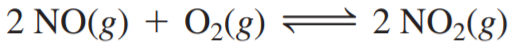×
Get Full Access to Chemistry: A Molecular Approach - 3 Edition - Chapter 14 - Problem 93e
Get Full Access to Chemistry: A Molecular Approach - 3 Edition - Chapter 14 - Problem 93e

×

# Consider the reaction: a. A reaction mixture at 175 KISBN: 9780321809247 1

## Solution for problem 93E Chapter 14

Chemistry: A Molecular Approach | 3rd Edition

• Textbook Solutions
• 2901 Step-by-step solutions solved by professors and subject experts
• Get 24/7 help from StudySoup virtual teaching assistantsChemistry: A Molecular Approach | 3rd Edition

4 5 1 348 Reviews
18
3
Problem 93E

Consider the reaction:a. A reaction mixture at 175 K initially contains 522 torr of NO and 421 torr of O2. At equilibrium, the total pressure in the reaction mixture is 748 torr. Calculate Kp at this temperature.

b. A second reaction mixture at 175 K initially contains 255 torr of NO and 185 torr of O2. What is the equilibrium partial pressure of NO2 in this mixture?

Step-by-Step Solution:

Solution 93EStep 1Equilibrium expression

Step 2 of 8

Step 3 of 8

##### ISBN: 9780321809247

The full step-by-step solution to problem: 93E from chapter: 14 was answered by , our top Chemistry solution expert on 02/22/17, 04:35PM. This full solution covers the following key subjects: torr, reaction, mixture, Equilibrium, initially. This expansive textbook survival guide covers 82 chapters, and 9454 solutions. This textbook survival guide was created for the textbook: Chemistry: A Molecular Approach, edition: 3. Chemistry: A Molecular Approach was written by and is associated to the ISBN: 9780321809247. Since the solution to 93E from 14 chapter was answered, more than 601 students have viewed the full step-by-step answer. The answer to “?Consider the reaction: a. A reaction mixture at 175 K initially contains 522 torr of NO and 421 torr of O2. At equilibrium, the total pressure in the reaction mixture is 748 torr. Calculate Kp at this temperature. b. A second reaction mixture at 175 K initially contains 255 torr of NO and 185 torr of O2. What is the equilibrium partial pressure of NO2 in this mixture?” is broken down into a number of easy to follow steps, and 68 words.

## Discover and learn what students are asking

Calculus: Early Transcendental Functions : Antiderivatives and Indefinite Integration
?In Exercises 7–10, complete the table to find the indefinite integral. Original Integral Rewrite I

Statistics: Informed Decisions Using Data : Scatter Diagrams and Correlation
?What does it mean to say two variables are positively associated? Negatively associated?

Statistics: Informed Decisions Using Data : Testing the Significance of the Least-Squares Regression Model
?In Problems 5–10, use the results of Problems 7–12, respectively, from Section 4.2 to answer the following questions: (a) What are the estima

Unlock Textbook Solution